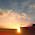## Thursday, February 3, 2011

### Bezier surface without using Evaluators OpenGl

Hi all,
This is my first post that contains some actual code. I wont provide the entire code for any of the posts, as it will be a spoon feeding. I will off-course give substantial part of it. (And to much extent with any optimizations, leaving them to the imagination of the user :))

We will use DeCasteljau Algorithm algorithm to draw the surface.The similar code using evaluators is available in Red Book Chapter 13.

Before going onto the code please learn a bit of opengl, as i will only explain how to calculate the points.

```GLfloat ctrlpoints;
//set the control points as u wish, 4 sets, 4 points each, x, y, z
int LOD=20;
//increase or decrease this (may be using +/-) to increase
number of curves making the surface
/* simple linear interpolation between two points
in three dimension*/
void lerp (GLfloat dest[], GLfloat a[], GLfloat b[], float t)
{
dest = a + (b-a)*t;
dest = a + (b-a)*t;
dest = a + (b-a)*t;
}

// evaluate a point on a bezier-curve. t goes from 0 to 1.0
void bezier (GLfloat dest[],GLfloat a[],GLfloat b[],
GLfloat c[],GLfloat d[], float t)
{
GLfloat ab,bc,cd,abbc,bccd;
lerp (ab, a,b,t);           // point between a and b
lerp (bc, b,c,t);           // point between b and c
lerp (cd, c,d,t);           // point between c and d
lerp (abbc, ab,bc,t);       // point between ab and bc
lerp (bccd, bc,cd,t);       // point between bc and cd
lerp (dest, abbc,bccd,t);   // point on the bezier-curve
}
/*
Given u and v, compute a point on the surface
*/
void eval(GLfloat dest[],float u,float v,GLfloat pnts)
{
GLfloat Q;
for(int i=0;i<4;i++)
{
bezier(Q[i],pnts[i],pnts[i],pnts[i],pnts[i],u);
}
bezier(dest,Q,Q,Q,Q,v);
}
/*
For orthogonal lines, change we need column vise points
This function find transpose of the matrix
*/
void get_vertical(GLfloat dest)
{
for(int i=0;i<4;i++)//ith column
{
for(int j=0;j<4;j++)//row
{
for(int k=0;k<3;k++)//elem
{
dest[i][j][k]=ctrlpoints[j][i][k];
}
}
}
}

void display()
{
/*
Intialization etc code here
*/

/*
Compute using algo
Plot them using GL_LINE_STRIP to get a smooth line, instead of discrete points
*/
for(int i=0;i<LOD;i++)
{
float u = (float)i/(LOD-1);
glBegin(GL_LINE_STRIP);
for(int j=0;j<LOD;j++)
{
float v = (float)j/(LOD-1);
GLfloat p;
eval(p,u,v,ctrlpoints);

glVertex3fv(p);

}
glEnd();
}

GLfloat dest;
get_vertical(dest);

for(int i=0;i<LOD;i++)
{
float u = (float)i/(LOD-1);
glBegin(GL_LINE_STRIP);
for(int j=0;j<LOD;j++)
{
float v = (float)j/(LOD-1);
GLfloat p;
eval(p,u,v,dest);

glVertex3fv(p);

}
glEnd();
}
/*
Swapping buffer etc code goes here

*/
}
```

1.Could you at least provide the initialization code for display() ?

2.@ecem you can find the initialization code in any introductory lesson to OpenGL. Just go through them once.

3.I am aware of these.. But when I use e.g

glClear(GL_COLOR_BUFFER_BIT | GL_DEPTH_BUFFER_BIT);
glPushMatrix();

for initialization and

glPopMatrix();
glFlush();

for the end, I got nothing but a black screen. Therefore I asked.

4.Alright, we made it work :) but how about a filled surface, changing GL_LINE_STRIP to GL_QUADS does not make the trick.

5.Unfortunately I am out of touch with OpenGl right now. I can pass on the code samples, that I used if u want.

1.This comment has been removed by the author.